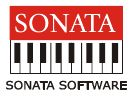# Placement Papers - SonataSONATA PLACEMENT PAPER (TECHNICAL)
Posted by :
Sushma
(31)
PAPER: SONATA PLACEMENT PAPER (TECHNICAL)

1. Full form of TTL and CMOS

2. Which is a good conductor (Extrinsic or Intrinsic)

3. What are the different types of capacitors (Electrolytic, dielectric...etc)

4. Select a passive component from the following (four choices were there)

5. Minimum no. of lines required for communication using RS232 (Ans:i think its 2)

6. To convert 1\'s complement to 2\'s complement and vice versa

7. During which time we use \'size of\' command. (ans: runtime)

8. Out of four choice we have to identify which is a macro.

9. There was one pointer _expression related question.

10. To find post fix _expression.

11. What type of operating system is unix (ans: pre-emptive,[not sure])

a)pre-emptive b)non-preemptive c)batch

12. Defnition of turing machine.

13. Where we use DFD(Data flow design)

a)structural languages b)object oriented languages c)UML d)all of the above

14. Name the error which occurs when we write on a page

a) segment fault b)permission fault c) page fault

15. A question based on the representation of an array in C. An array whose elements are fn pointers which returns a character

16. Point out error, if any, in the following program

main()
{ int i=1; switch(i)
{ case 1: printf("
break;
case 1*2+4:
printf("
Bottle for rent -inquire within"); break; }
Ans. No error. Constant expression like 1*2+4 are acceptable in cases of a switch.

17. Point out the error, if any, in the following program main()

{ int a=10,b; a>= 5 ? b=100 : b=200; printf("
%d",b); }

Ans. lvalue required in function main(). The second assignment should be

written in parenthesis as
follows:

a>= 5 ? b=100 : (b=200);

18. In the following code, in which order the functions would be called?
a= f1(23,14)*f2(12/4)+f3();
a) f1, f2, f3 b) f3, f2, f1
c) The order may vary from compiler to compiler d) None of the above

19. What would be the output of the following program?
main()
{ int i=4; switch(i)
{ default:
printf("
A mouse is an elephant built by the Japanese");
case 1:
printf(" Breeding rabbits is a hair raising experience");
break;
case 2:
printf("
Friction is a drag");
break;
case 3:
printf("
If practice make perfect, then nobody\'s perfect"); }
a) A mouse is an elephant built by the Japanese b) Breeding rabbits is a
hare raising experience
c) All of the above d) None of the above

20. What is the output of the following program?
#define SQR(x) (x*x)
main()
{ int a,b=3; a= SQR(b+2); printf("%d",a); }

a) 25 b) 11 c) error d) garbage value

21. In which line of the following, an error would be reported?

1. #define CIRCUM(R) (3.14*R*R);
2. main()
3. {
4. float r=1.0,c;
5. c= CIRCUM(r);
6. printf("
%f",c);
7. if(CIRCUM(r))==6.28)
8. printf("
Gobbledygook");
9. }
a) line 1 b) line 5 c) line 6 d) line 7

22. What is the type of the variable b in the following declaration?

#define FLOATPTR float*

FLOATPTR a,b;

a) float b) float pointer c) int d) int pointer

23. In the following code;

#include

main()

{ FILE *fp; fp= fopen("trial","r"); }

fp points to:

a) The first character in the file.

b) A structure which contains a "char" pointer which points to the first

character in the file.

c) The name of the file. d) None of the above.

24. We should not read after a write to a file without an intervening call

to fflush(), fseek() or rewind()

< TRUE/FALSE>

Ans. True

25. If the program (myprog) is run from the command line as myprog 1 2 3 ,

What would be the output